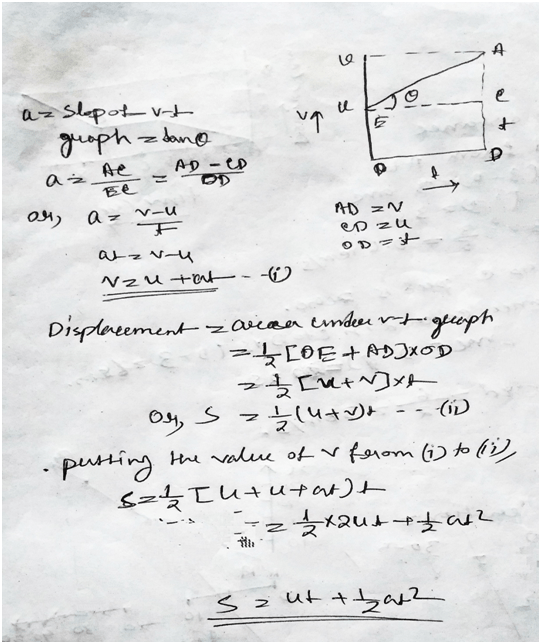# Chhattisgarh State Class 9 Science Chapter 4 Solution

Chhattisgarh State Board Class 9 Science Chapter 4 Atomic Structure Exercise Multiple Choice, Fill in the Blanks, Questions and Answers here.

## Chhattisgarh State Class 9 Science Chapter 4 Solution

1) Choose the right option-

(i) If the distance covered by a body is s=at + 1/2bt^2, then the acceleration of the body willbe-

(a) a (b) b (c) 2 b (d) a + b.

Ans: – option (b) b.

(ii) The area covered by velocity-time graph on the time axis is-

(a) m (b) m/s (c) m/s2 (d) None of these.

Ans: – option (c) m/s^2.

(iii) Below is the distance-time graph for the two bodies Aand B. Say which body has more velocity.

(a) A (b) B

(c) A and B (d) None of these.

Ans: – option (b) B.

(iv) Which one of the following is an example of uniform circular motion.

(a) Motion of earth around the sun.

(b) Motion of a toy train along a linear path.

(c) Motion of the second’s hand of a clock.

(d) Motion of a racing car along circular track.

Ans: – option (d).

2) Fill in the following blanks-

(i) A body is moving at a constant velocity; its acceleration will be ………..zero………….

(ii) The direction of velocity of a body depends on velocity rather than acceleration…………..

(iii) If the speed of an object is 72 km/h, its speed in m/s is…………20 m/s………

(iv) A person covers one round of a circular track of 2 m. radius in 2 s. What will be hisdisplacement after 8 second?

Ans: – Here displacement is zero. As he came back to the same point.

3) What is the meaning of a uniform circular motion? Give its two examples.

Ans: – If a body with uniform speed circulate any circular path, then this motion of the body is known as the circular motion. Example: – the cycle moving in circular path, satellite.

4) A body moves around the sun at a constant velocity. Is its motion a uniform motion or a non-uniform motion?

Ans: – The body moves around the sun at constant velocity is the example of uniform motion.

5) The displacement-time graph for an object is given below. What result can you find out for itsvelocity?

Ans: – From the graph we can clearly see that the ratio of displacement and time are constant in other word the acceleration is constant.

6) The position-time graph for a given object is a straight line parallel to its time axis. What can you sayabout its motion?

Ans: – The position time graph for a given object is a straight line parallel to itstime axis. So, by seeing this graph we can say that the object must be in rest.

7) Define uniform motion and a non-uniform motion also state two examples of each.

Ans: – The ratio of equal distance in proper time difference isknown as the uniform motion. The earth revolution is comparable to the uniform motion.

Whereas the ratio of unequal distance to the different time difference is known as the non-uniform motion. The motion of different vehicle in road in traffic.

8) Write all the three equations of motion and explain the symbols used in them.

Ans: –  The equations of motion are,

v=u + at;

s=ut + 1/2at^2;

V^2 = u^2 + 2as;

Here v= final velocity; u=initial velocity; a=acceleration; s=distance covered; t=time;

9) An athlete covers one round of a circular track of 7 m. radius is 44 sec. How much distance will itcover in 1 minute?

Ans: – The distance covered by athletes is = 2πr = 2 ×22/7×7^2 = 44 m.

The velocity is =44/44 = 1 s.

Then the total distance covered by athletes in 1 min is (60×1 =60) 60m.

10) Using the graphical method derive the second equation ofmotion s = ut + ½ at2.

Ans: –11) Neeraj reaches school in a car at an average speed of 20 km/h. While returning; his speed becomes30 km/h due to lesser crowd. What is the average speed of Neeraj’s car for the whole journey?

Ans: – The average speed = total distance / total time.

Let the distance to school is D; total time = D/20 + D/30;

So, average speed is = 2D/ (D/20 + D/30) = 120/5 =24 m/s.

12) A ball is dropped from the top of a 20 m. high tower. If its velocity is increasing with an acceleration of 10 m/s2then at what speed will the ball hit the ground? How much time will it take to hit the ground?

Ans: –13) A car is moving on a straight road at a uniform acceleration. The velocity of the car at different timesis given below. Draw its velocity-time graph and calculate its acceleration and also find out thedistance covered by it in 30 seconds.

t Time (s): – 0 10 20 30 40 50

v speed (m/s): – 5 10 15 20 25 30

Ans: –14) In the following figure, the position-time graph for an object at different times is shown. Calculate the speed of the object.

(i) A to B (ii) B to C (iii) C to B.

Ans: –15) A train starts moving from rest and acquires a velocity of 72 km/h in 5 minutes. If the train moves ata constant acceleration, calculate the following-

(i) Acceleration.

(ii) Distance travelled by the bus to acquire this velocity.

Ans: –

Let acceleration is a and distance travelled by bus is s.

Here initial velocity u=0;

Velocity=72 km/h = 72×5/18 = 20m/s.

Time is t= 5×60 = 300s.

As v=u + at;

Here a=? u=0; t=300s;

Then, 20 = 0+ 300a;

Or, a=1/15 = 0.06 m/a^2;

And, s=ut + 1/2at^2;

So, s= 0 + ½×0.06×(300)^2 ;

Or, s=3000m.

Updated: January 6, 2022 — 1:41 pm